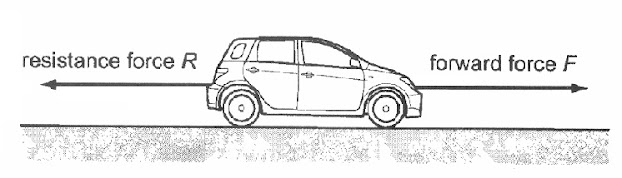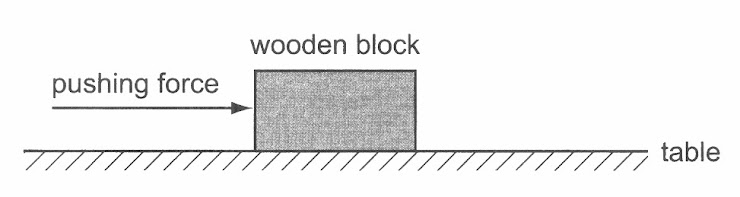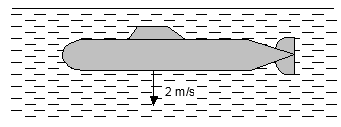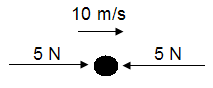Chapter 3 Forces Part 1 - Newton's First Law
• apply Newton's Laws to:
- describe the effect of balanced and unbalanced forces on a body
- describe the ways in which a force may change the motion of a body

Newton's first law of motion - sometimes referred to as the law of inertia. An object at rest stays at rest and an object in motion stays in motion with the same speed and in the same direction unless acted upon by an unbalanced force
Name *
Class *
Index Number *
Which is Newton's First Law of Motion? *
1 point
The net force acting on an object is zero. Which of the following statements are true? (X) The object may be at rest. (Y) The object may be travelling with constant velocity. (Z) The object may travelling with an acceleration. *
1 point
A car is moving forward. Two forces act on the car as shown. When force F equals force R, which statement is correct? *
1 pointA wooden block is pushed across a table at constant speed. Which statement is correct? *
1 pointA small submarine of mass 1000 kg sinks in water with a uniform speed of 2 m/s. What is the resultant force exerted on the submarine as it sinks? *
1 pointA particle moving at constant speed of 10 m/s is being acted on by two 5 N forces as shown. The particle will *
1 point